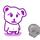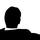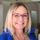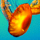Social QuestionHow do you calculate the power output of 238Pu over 25 years?

Asked by dr34m3r (308) February 13th, 2010

according to wikipedia:

“Most RTGs use 238Pu which decays with a half-life of 87.7 years, therefore diminish in power output by 1 − 0.51 / 87.7 or 0.787% of their capacity per year23 years after production, such an RTG will have decreased in power by 1 − 0.523 / 87.7 or 16.6%, i.e. providing 83.4% of its initial output. Thus, with a starting capacity of 470 W, after 23 years it would have a capacity of 0.834 * 470 W = 392 W.”

however, a more reliable source, states that the half life is 86.41 years….

how does one calculate the power output over 25 years?

i’m dying…

Observing members: 0Composing members: 0It follows the standard formula for exponential decay:

y = (initial amount) * 0.5 ^ (number of years / half-life value)

You can graph that equation as y = 0.5^(x/87.7) in your example and plug in 25 years to get the specific value:

y = 0.5^(25/87.7)
y = 0.82

So 82% of the original power output.

Feel free to play around with the formula in Wolfram Alpha (I’ve shortened the URL so it doesn’t break and taken the liberty to pre-filled in your example): http://bit.ly/c7Ft7V

timtrueman (5748)“Great Answer” (1) Flag as…@timtrueman GA.

but one more thing, how does one calculate the power output from each decay?

apparently 238Pu uses alpha decay e.e

dr34m3r (308)“Great Answer” (0) Flag as…is this a homework question?

Judi (39824)“Great Answer” (0) Flag as…Each year it decays by a percentage so the absolute power output drop changes year to year.

y = 0.5^(1/87.7)
y = 0.99212753…

So each year it becomes 99.21% of the previous year’s output.

For example in the first year on a 100 watt RTG power would decrease by 0.787 watts but in the 25th year power would decrease by only 0.651 watts (total power output goes from 82.7218 watts to 82.0706).

Alpha decay is some physics thing about how it physically decays. That’s not really my field of expertise unfortunately. But don’t confuse it with exponential decay.

timtrueman (5748)“Great Answer” (1) Flag as…@timtrueman

thank you so much.

i’m an idiot lol.

overthinking it.

i thought i had to do some mass defect and alpha particle calculation LOL XD

i had what you said written down on a paper, but i scrapped it cause i got worried about the watts and whatnot.

thank you.

dr34m3r (308)“Great Answer” (0) Flag as…You wre asking about alpha decay. It has no real bearing on your power output question and the exponential decay formula that @timtrueman gave you will give you the correct answer.

FYI: Alpha decay is a process by which a nucleus ejects a particle consisting of two protons and two neutrons. This is equivalent to a doubly-ionized Helium atom (no electrons). Because of it’s relatively large physical size and double ionization, the alpha particle is stopped very quickly and the resulting decay heat easily collected without requiring large amounts of heavy shielding to protect people.

Some RTGs also use beta-emitters such as Strontium-90. The effective end-of-life of an RTG occurs when it’s power output drops below the minimum power requirement of the device load.

If you need more information on the technical aspects of RTGs, just PM me.

stranger_in_a_strange_land (18285)“Great Answer” (0) Flag as…